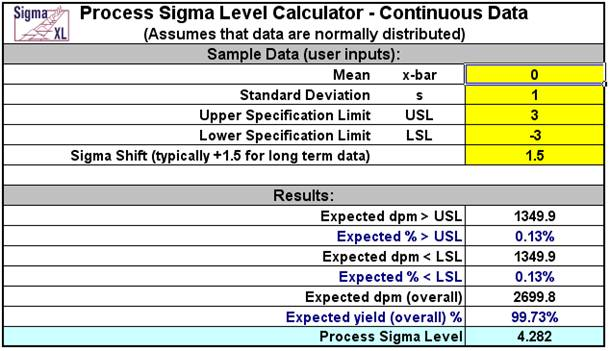Include Top

# Process Sigma Level Calculators

## Process Sigma Level – Discrete Data Example

Click SigmaXL > Templates & Calculators > Basic Process Capability Templates > Process Sigma Level – Discrete to access the Process Sigma Level – Discrete calculator. The template gives the following default example.Notes for use of the Process Sigma Calculator for Discrete Data:
1. Total number of defects should include defects made and later fixed.
2. Sample size should be large enough to observe 5 defects.

### Process Sigma Level – Continuous Data Example

Click SigmaXL > Templates & Calculators > Basic Process Capability Templates > Process Sigma Level – Continuous to access the Process Sigma Level – Continuous calculator. The template gives the following default example.Note: This calculator assumes that the Mean and Standard Deviation are computed from data that are normally distributed.

# Web Demos

Our CTO and Co-Founder, John Noguera, regularly hosts free Web Demos featuring SigmaXL and DiscoverSim